## MaplePrimes Posts

### Latest Post Latest Posts Feed

by: Maple V

The rocket fliesБыльнов_raketa_letit.mws

by:

Plotting the function of a complex variablePlotting_the_function_of_a_complex_variable.mws

by: Maple V3d_matryoshkas_en.mws

## Pearson correlation

Maple 2018

With this application developed entirely in Maple using native syntax and embedded components for science and engineering students. Just replace your data and you're done.

Pearson_Coeficient.mw

Lenin Araujo Castillo

## Foucault’s Pendulum Exploration Using MAPLE18

by: Maple 18

Foucault’s Pendulum Exploration Using MAPLE18

https://www.ias.ac.in/describe/article/reso/024/06/0653-0659

In this article, we develop the traditional differential equation for Foucault’s pendulum from physical situation and solve it from
standard form. The sublimation of boundary condition eliminates the constants and choice of the local parameters (latitude, pendulum specifications) offers an equation that can be used for a plot followed by animation using MAPLE. The fundamental conceptual components involved in preparing differential equation viz; (i) rotating coordinate system, (ii) rotation of the plane of oscillation and its dependence on the latitude, (iii) effective gravity with latitude, etc., are discussed in detail. The accurate calculations offer quantities up to the sixth decimal point which are used for plotting and animation. This study offers a hands-on experience. Present article offers a know-how to devise a Foucault’s pendulum just by plugging in the latitude of reader’s choice. Students can develop a miniature working model/project of the pendulum.

## Vector Force in space 3d

Maple 2018

Exercises solved online with Maple exclusively in space. I attach the explanation links on my YouTube channel.

Part # 01

Part # 02

Part # 03

Part # 04

Part # 05

Vector_Exercises-Force_in_space.mw

Lenin AC

## Algorithms

by: Maple

There are no efficient algorithms for this.
How would you simplify by hand the expression

```512*b^9 + (2303*a + 2304)*b^8 + (4616*a^2 + 9216*a + 4608)*b^7 + (5348*a^3 + 16128*a^2 + 16128*a + 5376)*b^6
+ (4088*a^4 + 16128*a^3 + 24192*a^2 + 16128*a + 4032)*b^5 + (1946*a^5 + 10080*a^4 + 20160*a^3 + 20160*a^2
+ 10080*a + 2016)*b^4 + (728*a^6 + 4032*a^5 + 10080*a^4 + 13440*a^3 + 10080*a^2 + 4032*a + 672)*b^3
+ (116*a^7 + 1008*a^6 + 3024*a^5 + 5040*a^4 + 5040*a^3 + 3024*a^2 + 1008*a + 144)*b^2
+ (26*a^8 + 144*a^7 + 504*a^6 + 1008*a^5 + 1260*a^4 + 1008*a^3 + 504*a^2 + 144*a + 18)*b + 9*a^8
+ 36*a^7 + 84*a^6 + 126*a^5 + 126*a^4 + 84*a^3 + 36*a^2 + 9*a + 1```

to  (a+2*b+1)^9 - a*(a-b)^8   ?

## Using the Lindstedt-Poincaré Method to Account...

Maple

We occasionally get asked questions about methods of Perturbation Theory in Maple, including the Lindstedt-Poincaré Method. Presented here is the most famous application of this method.

Introduction

During the dawn of the 20th Century, one problem that bothered astronomers and astrophysicists was the precession of the perihelion of Mercury. Even when considering the gravity from other planets and objects in the solar system, the equations from Newtonian Mechanics could not account for the discrepancy between the observed and predicted precession.

One of the early successes of Einstein's General Theory of Relativity was that the new model was able to capture the precession of Mercury, in addition to the orbits of all the other planets. The Einsteinian model, when applied to the orbit of Mercury, was in fact a non-negligible perturbation of the old model. In this post, we show how to use Maple to compute the perturbation, and derive the formula for calculating the precession.

In polar coordinates, the Einsteinian model can be written in the following form, where `u(theta)=a(1-e^2)/r(theta)`, with `theta` being the polar angle, `r(theta)` being the radial distance, `a` being the semi-major axis length, and `e` being the eccentricity of the orbit:

``````# Original system.
f := (u,epsilon) -> -1 - epsilon * u^2;
omega := 1;
u0, du0 := 1 + e, 0;
de1 := diff( u(theta), theta, theta ) + omega^2 * u(theta) + f( u(theta), epsilon );
ic1 := u(0) = u0, D(u)(0) = du0;
``````

The small parameter `epsilon` (along with the amount of precession) can be found in terms of the physical constants, but for now we leave it arbitrary:

```# Parameters.
P := [
a = 5.7909050e10 * Unit(m),
c = 2.99792458e8 * Unit(m/s),
e = 0.205630,
G = 6.6740831e-11 * Unit(N*m^2/kg^2),
M = 1.9885e30 * Unit(kg),
alpha = 206264.8062,
beta = 415.2030758
];
epsilon = simplify( eval( 3 * G * M / a / ( 1 - e^2 ) / c^2, P ) ); # approximately 7.987552635e-8```

Note that `c` is the speed of light, `G` is the gravitational constant, `M` is the mass of the sun, `alpha` is the number of arcseconds per radian, and `beta` is the number of revolutions per century for Mercury.

We will show that the radial distance, predicted by Einstein's model, is close to that for an ellipse, as predicted by Newton's model, but the perturbation accounts for the precession (42.98 arcseconds/century). During one revolution, the precession can be determined to be approximately

``sigma = simplify( eval( 6 * Pi * G * M / a / ( 1 - e^2 ) / c^2, P ) ); # approximately 5.018727337e-7``

and so, per century, it is `alpha*beta*sigma`, which is approximately 42.98 arcseconds/century.
It is worth checking out this question on Stack Exchange, which includes an animation generated numerically using Maple for a similar problem that has a more pronounced precession.

Lindstedt-Poincaré Method in Maple

In order to obtain a perturbation solution to the perturbed differential equation `u'+omega^2*u=1+epsilon*u^2` which is periodic, we need to write both `u` and `omega` as a series in the small parameter `epsilon`. This is because otherwise, the solution would have unbounded oscillatory terms ("secular terms"). Using this Lindstedt-Poincaré Method, we substitute arbitrary series in `epsilon` for `u` and `omega` into the initial value problem, and then choose the coefficient constants/functions so that both the initial value problem is satisfied and there are no secular terms. Note that a first-order approximation provides plenty of agreement with the measured precession, but higher-order approximations can be obtained.

To perform this in Maple, we can do the following:

``````# Transformed system, with the new independent variable being the original times a series in epsilon.
de2 := op( PDEtools:-dchange( { theta = phi/b }, { de1 }, { phi }, params = { b, epsilon }, simplify = true ) );
ic2 := ic1;```

```# Order and series for the perturbation solutions of u(phi) and b. Here, n = 1 is sufficient.
n := 1;
U := unapply( add( p[k](phi) * epsilon^k, k = 0 .. n ), phi );
B := omega + add( q[k] * epsilon^k, k = 1 .. n );```

```# DE in terms of the series.
de3 := series( eval( de2, [ u = U, b = B ] ), epsilon = 0, n + 1 );```

```# Successively determine the coefficients p[k](phi) and q[k].
for k from 0 to n do```

```    # Specify the initial conditions for the kth DE, which involves p[k](phi).
# The original initial conditions appear only in the coefficient functions with index k = 0,
# and those for k > 1 are all zero.
if k = 0 then
ic3 := op( expand( eval[recurse]( [ ic2 ], [ u = U, epsilon = 0 ] ) ) );
else
ic3 := p[k](0), D(p[k])(0);
end if:```

```    # Solve kth DE, which can be found from the coefficients of the powers of epsilon in de3, for p[k](phi).
# Then, update de3 with the new information.
soln := dsolve( { simplify( coeff( de3, epsilon, k ) ), ic3 } );
p[k] := unapply( rhs( soln ), phi );
de3 := eval( de3 );```

```    # Choose q[k] to eliminate secular terms. To do this, use the frontend() command to keep only the terms in p[k](phi)
# which have powers of t, and then solve for the value of q[k] which makes the expression zero.
# Note that frontend() masks the t-dependence within the sine and cosine terms.
# Note also that this method may need to be amended, based on the form of the terms in p[k](phi).
if k > 0 then
q := solve( frontend( select, [ has, p[k](phi), phi ] ) = 0, q );
de3 := eval( de3 );
end if;```

`end do:`

```# Final perturbation solution.
'u(theta)' = eval( eval( U(phi), phi = B * theta ) ) + O( epsilon^(n+1) );```

```# Angular precession in one revolution.
sigma := convert( series( 2 * Pi * (1/B-1), epsilon = 0, n + 1 ), polynom ):
epsilon := 3 * G * M / a / ( 1 - e^2 ) / c^2;
'sigma' = sigma;```

```# Precession per century.
xi := simplify( eval( sigma * alpha * beta, P ) ); # returns approximately 42.98``````

Maple Worksheet: Lindstedt-Poincare_Method.mw

## Solving a Numbrix Puzzle with Logic

by: Maple

Solving a Numbrix Puzzle with Logic

 Background Parade magazine, a filler in the local Sunday newspaper, contains a Numbrix puzzle, the object of which is to find a serpentine path of consecutive integers, 1 through 81, through a nine by nine grid.  The puzzle typically specifies the content of every other border cell.   The Maple Logic  package has a procedure, Satisfy , that can be used to solve this puzzle.  Satisfy is a SAT-solver; given a boolean expression it attempts to find a set of equations of the form, whereare the boolean variables in the given expression andare boolean values (true or false) that satisfy the expression (cause it to evaluate true).   A general technique to solve this and other puzzles with Satisfy is to select boolean-values variables that encode the state of the puzzle (a trial solution, whether valid or not), generate a boolean-expression of these variables that is satisfied (true) if and only if the variables are given values that correspond to a solution, pass this expression to Satisfy, then translate the returned set of boolean values (if any) to the puzzle solution.

Setup

Assign a matrix that defines the grid and the initial position.  Use zeros to indicate the cells that need values. To make it easy to inspect the expressions, a small 2 x 3 matrix is used for this demo---a full size example is given at the end.

 > M := Matrix(2,3, {(1,1) = 1, (1,3) = 5});(2.1)

Extract the dimensions of the Matrix

 > (m,n) := upperbound(M);(2.2)
 Boolean Variables Let the boolean variable x[i,j,k] mean that cell (i,j) has value k. For example, x[2,3,6] is true when cell (2,3) contains 6, otherwise it is false. There areboolean variables.

Initial Position

The initial position can be expressed as the following and-clause.

 > initial := &and(seq(seq(ifelse(M[i,j] = 0, NULL, x[i,j,M[i,j]]), i = 1..m), j = 1..n));(4.1)

The requirement that an interior cell with value k is adjacent to the cell with value k+1 can be expressed as the implication

x[i,j,k] &implies &or(x[i-1,j,k+1], x[i+1,j,k+1], x[i,j-1,k+1], x[i,j+1,k+1])

Extending this to handle all cells results in the following boolean expression.

 > adjacent := &and(seq(seq(seq(          x[i,j,k] &implies &or(NULL                                , ifelse(1(5.1)

All Values Used

The following expression is true when each integer, from 1 to, is assigned to one or more cells.

 > allvals := &and(seq(seq(&or(seq(x[i,j,k], k=1..m*n)), i=1..m), j=1..n));(6.1)

Single Value

The following expression is satisfied when each cell has no more than one value.

 > single := ¬ &or(seq(seq(seq(seq(x[i,j,k] &and x[i,j,kk], kk = k+1..m*n), k = 1..m*n-1), i = 1..m), j = 1..n));(7.1)

Solution

Combine the boolean expressions into a a single and-clause and pass it to Satisfy.

 > sol := Logic:-Satisfy(&and(initial, adjacent, allvals, single));(8.1)

Select the equations whose right size is true

 > sol := select(rhs, sol);(8.2)

Extract the lhs of the true equations

 > vars := map(lhs, sol);(8.3)

Extract the result from the indices of the vars and assign to a new Matrix

 > S := Matrix(m,n):
 > for v in vars do S[op(1..2,v)] := op(3,v); end do:
 > S;(8.4)
 Procedure We can now combine the manual steps into a procedure that takes an initialized Matrix and fills in a solution. Numbrix := proc( M :: ~Matrix, { inline :: truefalse := false } )

Example

Create the initial position for a 9 x 9 Numbrix and solve it.

 > P := Matrix(9, {(1,1)=11, (1,3)=7, (1,5)=3, (1,7)=81, (1,9)=77, (3,9)=75, (5,9)=65, (7,9)=55, (9,9)=53                , (9,7)=47, (9,5)=41, (9,3)=39, (9,1)=37, (7,1)=21, (5,1)=17, (3,1)=13});(10.1)
 > CodeTools:-Usage(Numbrix(P));
 memory used=0.77GiB, alloc change=220.03MiB, cpu time=15.55s, real time=12.78s, gc time=3.85s(10.2)
 >

numbrix.mw

## Maple 2019.1 update

by: Maple

We have just released an update to Maple, Maple 2019.1.

Maple 2019.1 includes corrections and improvement to the mathematics engine (including LPSolve, sum, statistics, and the Physics package),  visualization (including annotations and the Plot Builder), export to LaTeX (exporting output) and PDF (freezing on export), network licensing, MATLAB connectivity, and more.  We recommend that all Maple 2019 users install these updates.

This update is available through Tools>Check for Updates in Maple, and is also available from our website on the Maple 2019.1 download page, where you can also find more details.

## Maple Conference – New Deadline for Submissions

by: Maple , Maple Toolboxes

I just wanted to let everyone know that the Call for Papers and Extended Abstracts deadline for the Maple Conference has been extended to June 14.

The papers and extended abstracts presented at the 2019 Maple Conference will be published in the Communications in Computer and Information Science Series from Springer. We welcome topics that fall into the following broad categories:

• Maple in Education
• Algorithms and Software
• Applications of Maple

https://www.maplesoft.com/mapleconference/Papers-and-Presentations.aspx

Hope to hear from you soon!

## A finite difference scheme for the heat equation...

Maple
 I describe here a finite difference scheme for solving the boundary value problem for the heat equationfor the unknown temperaturesubject to the boundary conditionsand the initial conditionThis finite difference scheme is designed expressly with the goal of avoiding differentiating the conductivity, thereforeis allowed to be nonsmooth or even discontinuous. A discontinuousis particularly important in applications where the heat conduction takes place through layers of distinct types of materials.   The animation below, extracted from the worksheet, demonstrates a solution  corresponding to a discontinuous.  The limit of that solution as time goes to infinity, which may be calculated independently and exactly, is shown as a gray line.## MapleSim 2019 is now available!

We’re excited to announce that we have just released a new version of MapleSim. The MapleSim 2019 family of products helps you get the answers you need from your models, with improved performance, increased modeling scope, and more ways to connect to your existing toolchain. Improvements include:

• Faster simulation speeds, both within MapleSim and when using exported MapleSim models in other tools

• More simulation options are now available when running models imported from other systems

• Additional options for FMI connectivity, including support for variable-step solvers with imported FMUs, and exporting models using variable step solvers using the MapleSim FMI Connector add-on

• Improved interactive analysis apps for Monte Carlo analysis, Optimization, and Parameter Sweep

• Expanded modeling scope in hydraulics, electrical, multibody, and more, with new built-in components and support for more external Modelica libraries

• New add-on library: MapleSim Engine Dynamics Library from Modelon provides specialized tools for modeling, simulating, and analyzing the performance of combustion engines

• New add-on connector: The B&R MapleSim Connector gives automation projects a powerful, model-based ability to test and visualize control strategies from within B&R Automation Studio

It is a very good computational tool to perform modeling and simulation using our world as a reference. You can also teach math knowing how to choose the right icons.
I recommend this software to everyone who wants to simulate objects or multibodies. In any case, knowledge of physics and mathematics, especially vector mechanics, is necessary.
Very grateful to the Maplesoft company for sharing their projects through the MapleSim gallery.

From now on all projects will be with Maple and MapleSim.

Lenin AC

## macro vs. subs

Maple

macros can be made to work like subs, you just need to know a few tricks to get it to work the same way.  macros just works in a slightly different manner and we can make it useful.

The difference is with subs, one has to keep specifying the substitution with each equation you want subbed, whereas macro will already have it defined.  As an example:

a := v^2*z^3 - 34/(5*x^2*sin(y*v^2)) + 36*v^2 - b*v^2 + 3^(v^2 - cos(v^2 + g))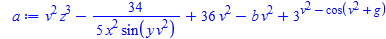If we want to substitute h for v^2, then we would normally do this using subs

subs(v^2=h,a)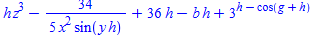however, we can also use macro

macro(v^2=h)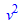now it doesn't just automatically substitute those values so we need to coax maple a little bit.  We can do that by converting the equation to a string and parsing it.

parse(convert(a,string))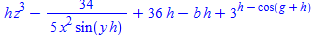so as you see we arrive at the same result.  Now there is a caveat using macro, if you've already defined a variable in a macro, subs will not work using the same variable sustitution - you first need to reset the variable in the macro back to itself.

subs(v^2=h,a)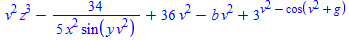#doesn't work since the variable is defined in a macro

macro(v^2=v^2) #reset the variable in the macro

subs(v^2=h,a)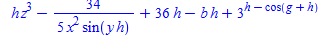# now it works

we could also define a little procedure to simplify our typing, to have the macro variable work on our equation.

mvs:=proc(a) #macro variable substitution
parse(convert(a,string));
end proc:

macro(v^2=h)
mvs(a)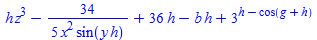now if we had some other existing equation before defining the macro
aa:=exp(v^2-sin(theta))+v^2*cos(theta)-1/x^sin(v^2-g)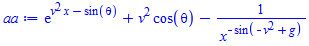we just have to simply apply our proc on the equation to apply the variable substitution
mvs(aa)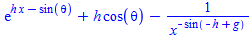﻿